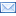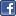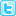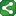## Saturday, June 27, 2009

### %EVAL AND %SYSEVALF MACRO FUNCTIONS: GETTING TO KNOW THEM BETTER.

With the exception of %sysevalf function, integer arithmetic is the only way macro statements perform arithmetic calculations.
Following are the few examples of macro statements performing integer arithmetic calculations:
%let one=%eval (3+5);
%let two=%eval (5*2);
%let three=%eval (9/3);
%let four=%eval (5/2);
%put The value of one is &one;
%put The value of two is &two;
%put The value of three is &three;
%put The value of four is & four;

Open the Log file and see the results as follows:
The value of one is 8
The value of two is 10
The value of three is 3
The value of four is 2
The value for macro variable four, should be 2.5, instead it shows only two. That happens because if we perform division on integers, integer arithmetic doesn’t take the fractional part into account.
When we try to execute the integer arithmetic calculations of values with functional part, :

%let last= %eval (5.0+3.0); /*INCORRECT*/

%EVAL function only supports integer arithmetic values. The values here in the above statement have a period character to numeric values and because of that the macro processor stops evaluating and produces the following error message: “ ERROR: A character operand was found in the %EVAL function or %IF condition where a numeric operand is required. The condition was: 5.0+3.0 “

So our next question comes is… how to perform these type of calculations:
Here comes %sysevalf to rescue you, because this function is capable of evaluating the floating point operands.

Evaluating Floating Point Operands
The %SYSEVALF function can perform arithmetic calculations with operands that have the floating point values.
Here are some of the examples where %SYSEVALF function becomes handy:
%let test= %sysevalf(1.0*3.0);
%let final= %sysevalf(1.5+2.8);
%let last= %sysevalf(5/3);
%put The value of test is &test;
%put The value of final is &final;
%put The value of last is &last;
The %PUT statements display the following messages in the log:
The value of test is 3
The value of final is 4.3
The value of last is 1.66666666666666
%SYSEVALF function perform arithmetic calculations and the result of the evaluation can be a floating point value like in the final and last macro variable case, but as in integer arithmetic calculations, the result is always a text.
The %SYSEVALF function be used in conjugation with other functions like, INTEGER, CEIL, and FLOOR.
For example, the following %PUT statements return 3, 4 and 3 respectively:
%let val=3.8;
%put %sysevalf(&val,integer);
*Value returns in the log is 3;
%put %sysevalf(&val, ceil);
*Value returns in the log is 4;
%put %sysevalf(&val,floor);
*Value returns in the log is 3;

Difference between %eval and %sysevalf functions can be understand better with the following example;
%let value=9;
%let value2=5;
%let newval=%sysevalf(&value/&value2);
%let newval1=%eval(&value/&value2);
%put &newval;
%put &newval1;
*Ans: newval=1.8;
*Ans: newval1=1;ShareThis